# How to correctly use I-Splines for monotone non-decreasing/ increasing regression?

I have the following data to which I want to fit a monotone non-decreasing spline.

x <- c(300, 377, 455, 533, 611, 688, 766, 844, 922, 1000)
y <- c(0.5, 0.8, 1, 1, 1, 1, 1, 1, 1, 1)


To achieve this, I use an I-Spline basis (i.e., splines2::iSpline) paired with non-negative coefficients (i.e., nnls::nnls).

# Inner knots.
k <- quantile(x, probs = c(.25, .5, .75))

h <- splines2::iSpline(x, knots = k, Boundary.knots = range(x), intercept = TRUE)
a <- nnls::nnls(h, y)$x  And this gives me the following: # Fitted. f <- h %*% a print(f) # [,1] # [1,] 0.0000000 # [2,] 0.8106201 # [3,] 0.9699174 # [4,] 0.9992354 # [5,] 1.0035000 # [6,] 1.0035515 # [7,] 1.0035515 # [8,] 1.0035515 # [9,] 1.0035515 # [10,] 1.0035515  However, with intercept = TRUE, I would expect the spline to start somewhere above the first data point. For instance, this seems to be the case when I set the first basis function to 1. h[, 1] <- 1  If I set intercept = FALSE, then things go haywire.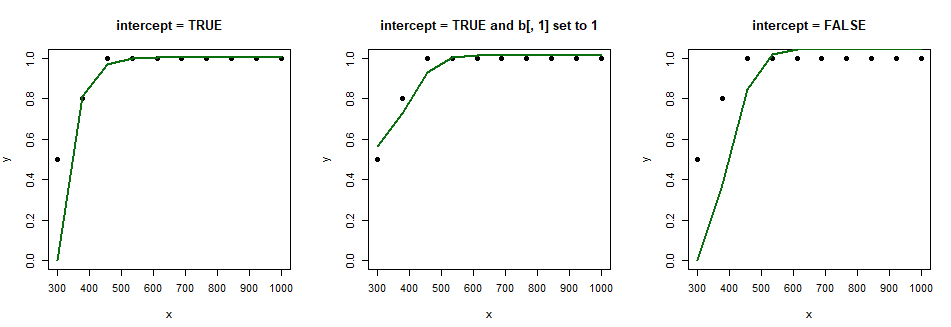Can you please help me understand what is going on, i.e., why is the spline not starting at (300, 0.5)? ### Further Details I want to clarify my question further. My confusion stems from what is written in this paper on p. 15: To get a basis for the increasing splines we need to add the constant function to the I-splines and allow it to enter the linear combination with either sign. In the splines2 package documentation, where the intercept argument for iSpline() is described, it says: Notice that when using I-Spline for monotonic regression, intercept = TRUE should be set even when an intercept term is considered additional to the spline bases in the model. What I get from this is that if I want to fit a monotone spline with, say, nnls::nnls(), then I need to add a constant function to the I-Spline basis, i.e., h <- cbind(1, h), assuming that h is the basis matrix output of iSpline(). However, in the paper linked above, that's not always the case. Sometimes the author replaces the first column of the basis matrix (i.e., h <- cbind(1, h[ -1]) with the constant function, and, other times, he appends the constant function to the basis matrix. For instance, if I create the I-Spline basis matrix as follows: h <- splines2::iSpline(x, knots = c(3, 5, 8), degree = 0, intercept = TRUE)  Then, depending on how I "add" the constant function (or not) I get different splines: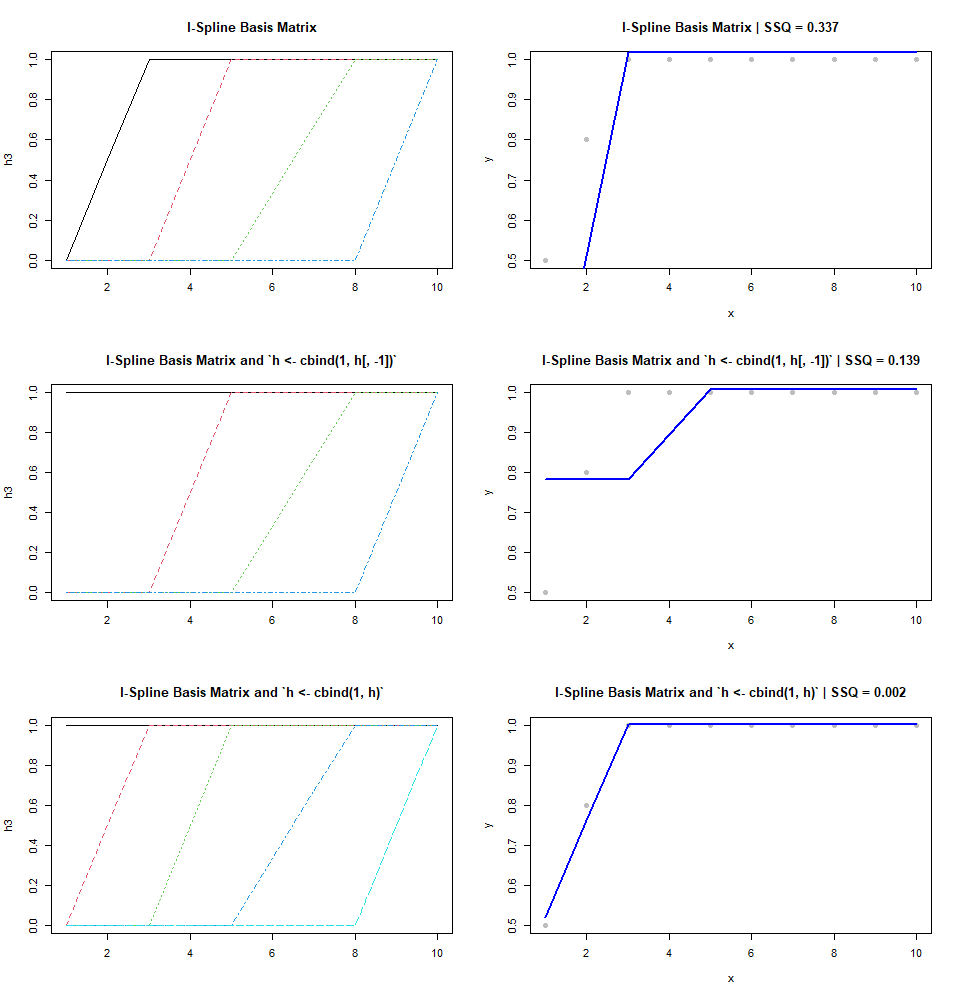What's not clear to me is how the basis matrix provided by iSpline() is intended to be used in this case. ## 2 Answers Looking back at this question after asking the author of the splines2 package for more details, I feel like I owe a clarification, which may also qualify as an answer. The following is based heavily on two GitHub issues that I opened here (#4) and here (#6). I am posting it here in case it might help someone in the future. In issue #4 I was confused because I didn't understand how exactly to use I-Splines in the context of regression. My goal was to use I-Splines to fit monotone non-increasing or non-decreasing splines. I found your package splines2 and the code of De Leeuw (2017) online and that helped to contrast and compare the approaches. I learned that splines2 creates I-Splines based on Ramsay (1988) who uses M-Splines as a starting point. On the other side, De Leeuw (2017) constructs I-Splines using N-Splines as a starting point. Both approaches are not approximate, but equivalent. So, I was confused when using the same configuration of knots I got different I-Splines. It turns out that both Ramsay (1988) and De Leeuw (2017) use in fact B-Splines, but normalized differently. Ramsay (1988) uses B-Splines that are normalized such that they integrate to one (i.e., these are called M-Splines). Whereas for De Leeuw (2017), the B-Splines are normalized such that they sum to one (i.e., which he calls normalized B-Splines, or N-Splines). Also, it appears that Ramsay (1988) refers to N-Splines simply as B-Splines in his paper. This seems to also be the case with splines2::bSpline() which actually returns normalized B-Splines that sum to one, i.e., N-Splines. Indeed, normalized B-Splines and M-Splines look the same, and we can see that they only differ with respect to the normalization (i.e., y axis). # The data. x = 1:10 # The inner knots. innerknots = c(5) # The degree. degree = 2 # Creating normalized B-Splines and M-Splines. b <- splines2::bSpline(x, knots = innerknots, degree = degree, intercept = TRUE) m <- splines2::mSpline(x, knots = innerknots, degree = degree, intercept = TRUE) # Plotting the spline bases. matplot(b, type = "l", lwd = 2, main = paste0("Normalized B-Splines | degree = ", degree)) matplot(m, type = "l", lwd = 2, main = paste0("M-Splines | degree = ", degree))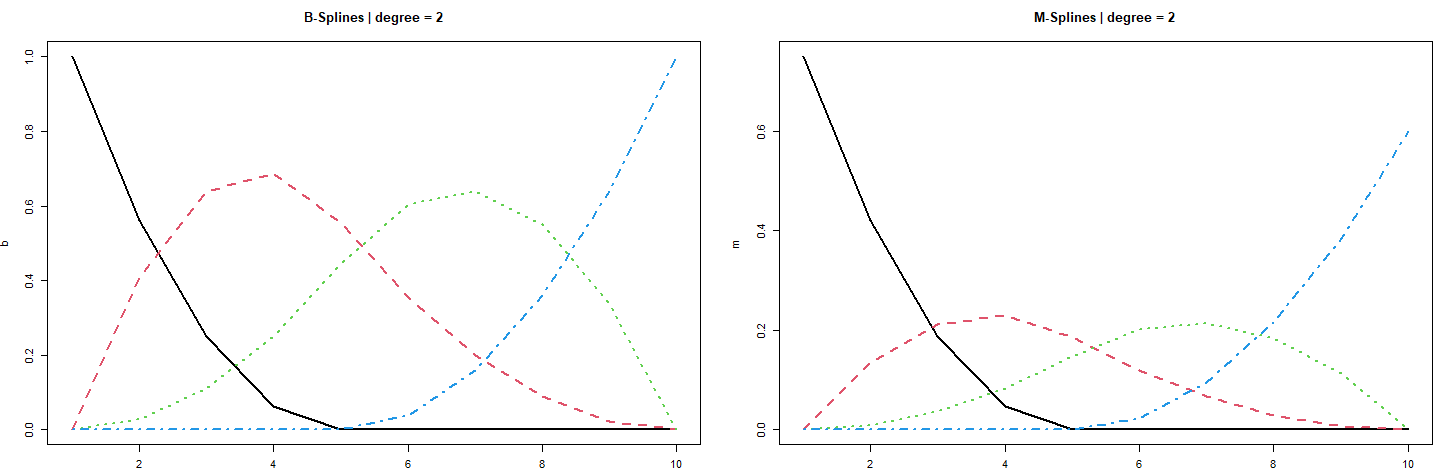Now, onto creating I-Splines, Ramsay (1988) says that they can be created by taking the integrals of M-Splines. Which is what the argument integral in splines2::mSpline() does, or what the function splines2::iSpline() provides. # Taking integrals of M-Splines and creating I-Splines. mi <- splines2::mSpline(x, knots = innerknots, degree = degree, intercept = TRUE, integral = TRUE) i <- splines2::iSpline(x, knots = innerknots, degree = degree, intercept = TRUE) # Plotting the spline basis. matplot(mi, type = "l", lwd = 2, main = paste0("Integrals of M-Splines | degree = ", degree)) matplot(i, type = "l", lwd = 2, main = paste0("I-Splines | degree = ", degree))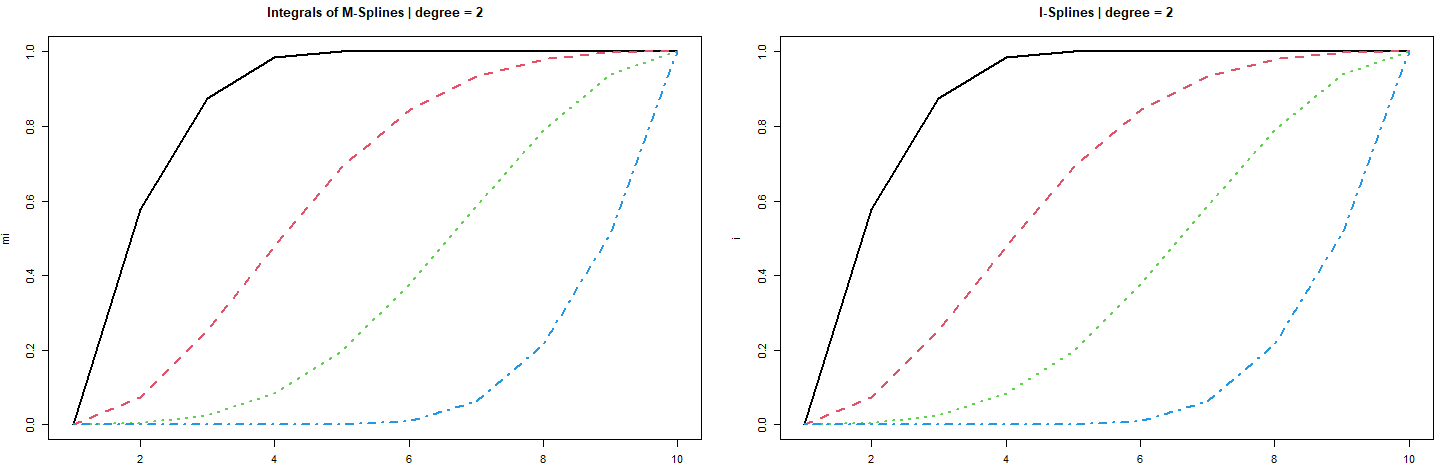De Leeuw (1988; p. 17) refers to a formula (see below) from De Boor (1976) and says: This shows that I-splines can be computed by using cumulative sums of B-spline values. To be more precise, I believe this should read as "sums of normalized B-spline values". $$\int_{-\infty}^{x} M_{j, m}(t) d t=\sum_{\ell=j}^{j+r} N_{\ell, m+1}(x)$$ There are two things to note. First, De Leeuw (2017) uses m to refer to the order of a spline while Ramsay (1988) refers to the degree. If I am not mistaken the relationship should be degree = order - 1. Second, and most importantly, the equation above shows that integrals of M-Splines of order m are equivalent to cumulative sums of normalized B-Splines of order m + 1. Indeed, it seems that by the equation above we can obtain the same I-Splines. So, above I used the splines2::iSpline() to create I-Splines of degree = 2. Thus, if I want to use cumulative sums to get the same I-Splines, I need normalized B-splines that are one degree higher, i.e., degree = 2 + 1. # Create normalized B-Splines of one degree higher. b2 <- splines2::bSpline(x, knots = innerknots, degree = degree + 1, intercept = TRUE) # Now take the cumulative sums as De Leeuw (2017) shows in the paper. # And remove the redundant column of zeros at the end of the basis matrix. ci <- 1 - t(apply(b2, 1, cumsum)) ci <- ci[, -ncol(ci)] # And, indeed, these are the same splines as those provided by splines2::iSpline(). all(round(i, 10) == round(ci, 10)) # Plot the spline bases. matplot(i, type = "l", lwd = 2, main = paste0("I-Splines | degree = ", degree)) matplot(ci, type = "l", lwd = 2, main = paste0("Cumulative sums of normalized B-Splines | degree = ", degree))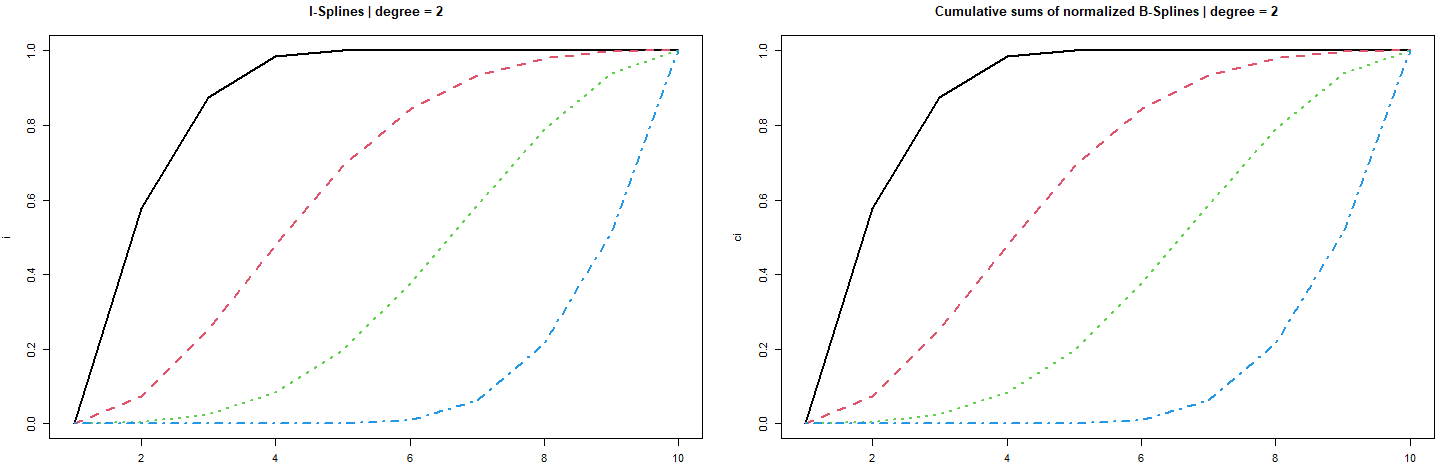I was happy when I saw this equivalence. My first confusion came from the fact that I overlooked the m + 1 part in the equation, and this sent me down the rabbit hole of chasing intercepts... Related to my second confusion, Ramsay (1988; p. 428) says: We shall use the term order k to refer to an M-spline of degree k - 1 or the associated I-spline of degree k. I tried to replicate this with splines2, but I couldn't observe the same. For example, I expected to be able to obtain the I-Splines above (i.e., stored in i), constructed with degree = 2, by taking integrals of M-Splines constructed with degree = 2 - 1. But that doesn't seem to be the case. # Create M-Splines of one degree lower. mspline <- splines2::mSpline(x, knots = innerknots, degree = degree - 1, intercept = TRUE, integral = TRUE) # Plotting the M-Splines. matplot(mspline, type = "l", lwd = 2, main = paste0("Integrals of M-Splines | degree = ", degree)) # But the I-Splines with degree = 2 look like: matplot(i, type = "l", lwd = 2, main = paste0("I-Splines | degree = ", degree))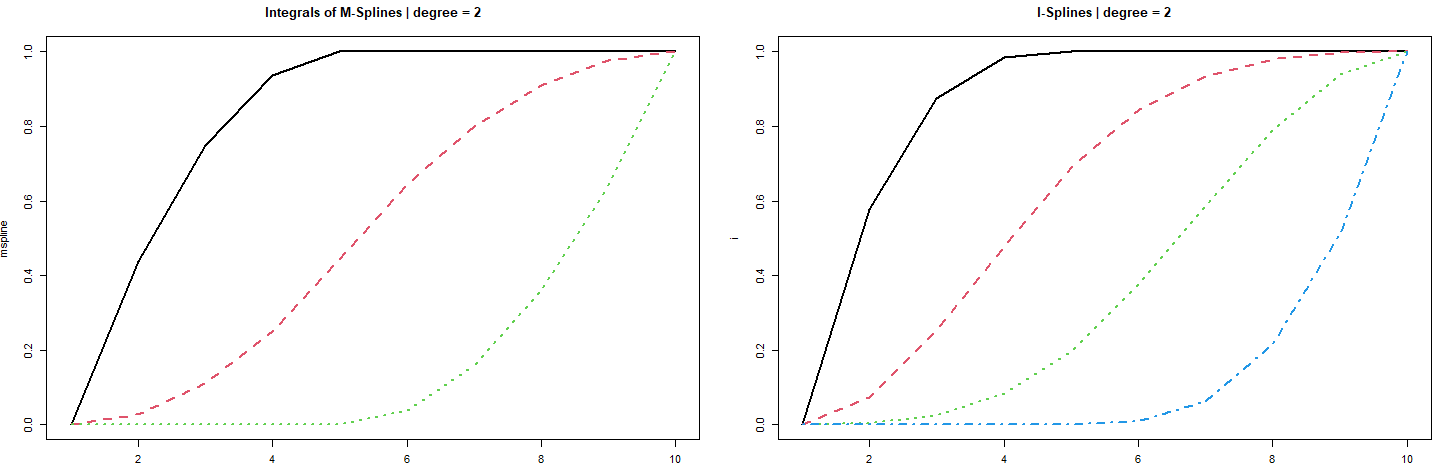The author of splines2 clarifies this in #6 and he points me to the documentation that reads: The degree of I-spline defined to be the degree of the associated M-spline instead of actual polynomial degree. For example, I-spline basis of degree 2 is defined as the integral of associated M-spline basis of degree 2. Based on this piece of information and the equation above that links integrals of M-Splines with cumulative sums of N-Splines, it becomes clear that if I want an I-Spline basis of degree = 3, then I can obtain this from integrals of M-Splines of degree = 2, which will be identical to cumulative sums of N-Splines of degree = 3. And, indeed this seems to be the case: # Creating normalized B-Splines of degree 3. nspline <- splines2::bSpline(x, knots = innerknots, degree = 3, intercept = TRUE) # Computing I-Splines as cumulative sums of normalized B-Splines. cumsum_nspline <- 1 - t(apply(nspline, 1, cumsum)) cumsum_nspline <- cumsum_nspline[, -ncol(cumsum_nspline)] # Computing integrals of M-Splines of degree 2. integrals_mspline <- splines2::mSpline(x, knots = innerknots, degree = 2, intercept = TRUE, integral = TRUE) # Computing I-Splines of degree 2 associated with the M-Splines. ispline <- splines2::iSpline(x, knots = innerknots, degree = 2, intercept = TRUE) # Plotting the spline bases. matplot(cumsum_nspline, type = "l", lwd = 2, main = paste0("Cumulative Sums Normalized B-Splines | degree = ", 2)) matplot(integrals_mspline, type = "l", lwd = 2, main = paste0("Integrals M-Splines | degree = ", 2)) matplot(ispline, type = "l", lwd = 2, main = paste0("I-Splines w/ M-Spline associated degree | degree = ", 2))To come full circle, my third confusion was related to how the I-Spline basis should properly be used for monotone regression. I noticed that, despite the fact that De Leeuw (2017) provides the I-Spline basis with the constant function already appended as the first basis function, at one point he drops the first basis function and appends another column of ones (i.e., g <- cbind (1, h[,-1])). This looks odd, but it seems to be a choice given the data he was modeling. Neverthless, in the paper De Leeuw (2017, p.15) states: Non-negative linear combinations I-splines can be used as a basis for the convex cone of increasing splines. Note, however, that if we use an extended partition, then all I-splines start at value zero and end at value one, which means their convex combinations are the splines that are also probability distributions on the interval. To get a basis for the increasing splines we need to add the constant function to the I-splines and allow it to enter the linear combination with either sign. Thus, it seems that to properly uses I-Spline bases for fitting monotone non-decreasing or non-increasing splines, one needs to: 1. add a constant function to the I-Spline basis 2. leave the first parameter unconstrained (i.e., the parameter associated with the constant function; the intercept) 3. constrain all other parameters to be non-negative (i.e., for a monotone non-decreasing spline) or non-positive (i.e., for a monotone non-increasing spline) There are different approaches to estimate given such constraints, but, as Tom Wenseleers points in his answer (i.e., also see the edits for his answer), one can express the problem as a quadratic programming problem and use quadprog or osqp. For instance, using quadprog::solve.QP() this might look like: # Create I-Spline basis. ispline <- splines2::iSpline(x, knots = innerknots, degree = 2, intercept = TRUE) # Add constant to I-Spline basis. ispline <- cbind(1, ispline) # Fit monotone non-decreasing spline for increasing toy data. y_increase <- c(-0.2, 0.3, 0.5, 0.7, 0.6, 1, 0.9, 1, 1, 1) # Creating D matrix and d vector. d_mat <- crossprod(ispline, ispline) d_vec <- crossprod(ispline, y_increase) # Setting equality constraints s.t., all paramters are >= 0. a_mat <- diag(1, ncol(ispline)) b_vec <- rep(0, ncol(ispline)) # Freeing the first parameter. a_mat[1, 1] <- 0 # Solving the problem. alpha_non_negative <- solve.QP(Dmat = d_mat, dvec = d_vec, Amat = t(a_mat), bvec = b_vec)$solution

# Getting fitted values (i.e., the spline).
spline_non_decreasing <- ispline %*% alpha_non_negative


The same goes for a monotone non-increasing spline, but this time with non-positive constraints:

# Reusing the I-Spline stored in ispline.

# Fit monotone non-increasing spline for decreasing toy data.
y_decrease <- rev(y_increase)

# Creating D matrix and d vector.
d_mat <- crossprod(ispline, ispline)
d_vec <- crossprod(ispline, y_decrease)

# Setting equality constraints s.t., all paramters are <= 0.
a_mat <- diag(-1, ncol(ispline))
b_vec <- rep(0, ncol(ispline))

# Freeing the first parameter.
a_mat[1, 1] <- 0

# Solving the problem.
alpha_non_positive <- solve.QP(Dmat = d_mat, dvec = d_vec, Amat = t(a_mat), bvec = b_vec)\$solution

# Getting fitted values (i.e., the spline).
spline_non_increasing <- ispline %*% alpha_non_positive


Which results in the following splines:

plot(x, y_increase, pch = 19, cex = 1.5, col = "darkgray", main = paste0("Non-decreasing | SSQ = ", round(sum((y_increase - spline_non_decreasing)^2), 5)), cex.main = 2)
lines(x, spline_non_decreasing, lwd = 2, col = "rosybrown")
points(x, spline_non_decreasing, pch = 19, cex = 1.5, col = "steelblue")

plot(x, y_decrease, pch = 19, cex = 1.5, col = "darkgray", main = paste0("Non-increasing | SSQ = ", round(sum((y_decrease - spline_non_increasing)^2), 5)), cex.main = 2)
lines(x, spline_non_increasing, lwd = 2, col = "rosybrown")
points(x, spline_non_increasing, pch = 19, cex = 1.5, col = "steelblue")I hope this makes things a bit more clear.

From the definition of k in your code we know the first segment is fitting more than 2 data points.

Only if it is fitting first 2 data points and without the constraint that the function to be continuous, the spline will pass the first point. Note, if that is the case, the model is not a spline.

library(ggplot2)
x <- c(300, 377, 455, 533, 611, 688, 766, 844, 922, 1000)
y <- c(0.5, 0.8, 1, 1, 1, 1, 1, 1, 1, 1)
group <- c(0,0,1, 1, 1, 1, 1, 1, 1, 1)
d = data.frame(x=x,y=y, group=group)
ggplot(d,aes(x,y, group=group))+geom_point()+geom_smooth(method='lm')+theme_gray(20)• Haitao Du, what you wrote makes sense. However, I am still confused because in this paper on p. 15 (last sentence), it says that a constant function needs to be added to the I-Spline basis matrix. However, in the paper, the first column of the basis matrix is sometimes replaced with ones (i.e., h <- cbind(1, h[ -1]) and other times simply another column is appended (i.e., h <- cbind(1, h)). Can you please shed some light on why this is the case? Apr 20, 2021 at 8:59
• I will append this to the question above. Apr 20, 2021 at 9:15